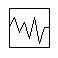# Noise Controller

(Redirected from Controller -- Noise)

Navigation: Models ➔ Control 2 Models ➔ Noise Controller

## General Description

• This unit operation is used to simulate process noise. It can be used to add disturbance to Feeders, pipe outlet capacity and other writeable variables.
• The Noise Control model is an orphan model, i.e it cannot be connected to any other unit operation.
• The noise model is for dynamic simulation only with the purpose of adding disturbance to the model.

### DiagramThe diagram shows the default drawing of the Noise control unit.

## Inputs and Outputs

There are no connections to this unit.

## Model Theory

The random number generator used for all probability functions is from the C++ standard library (http://www.cplusplus.com/reference/random/).

The Probability Density functions used for the Noise generation are shown in the following table. In all cases the following convention is used:

$\displaystyle{ \mu }$ = Mean Value
$\displaystyle{ \sigma }$ = Standard Deviation

For all methods, the tag PlantModel.RandomSeedAtStart (set on the Plant Model - FlwSolve page) affects the repeatability of the random values generated. For the same seed value, a repeatable set of random numbers will be generated. For a different seed, a different set of random numbers will be generated. If "*" is used, then a new random set of values will be generated on every run.

Type Probability Density Function P(t) NOTES
Gaussian
• $\displaystyle{ P(t) = \cfrac{1}{\sigma \sqrt{2\pi}}\cdot e^{\dfrac{-(t-\mu)^2}{2\sigma^2}} }$
where $\displaystyle{ \sigma \gt 0 }$
Flat
• $\displaystyle{ P(t) = 0 }$
where $\displaystyle{ t \lt \mu - m }$ or $\displaystyle{ t \gt \mu + m }$
• $\displaystyle{ P(t) = \dfrac{1}{2\sigma} }$

where $\displaystyle{ \mu - m ≤ t ≤; \mu + m }$ and $\displaystyle{ m ≥; 0 }$ and $\displaystyle{ m }$ = maximum deviation

Poisson
• $\displaystyle{ P(t) = \cfrac{\mu^t}{t!}\ e^{-\mu} }$
where $\displaystyle{ e }$ = the base of the natural logarithm system (2.71828...) and $\displaystyle{ \mu \gt 0 }$
Gamma
• $\displaystyle{ P(t) = \cfrac{1}{\Gamma(\alpha)\ \beta^{\alpha}}\ t^{\alpha-1}\ e^{-t/\beta} }$

where $\displaystyle{ \alpha ≥ 0.5 }$ and $\displaystyle{ \beta \gt 0 }$

Note: The Chi-squared distribution is a special case of the Gamma distribution
with $\displaystyle{ \alpha = n/2 }$ and $\displaystyle{ \beta = 2 }$, where $\displaystyle{ n }$ = degrees of freedom

Weibull
• $\displaystyle{ P(t) = \alpha^{-\beta}\ \beta\ t^{\beta-1}\ e^{-(t/\alpha)^\beta} }$

where $\displaystyle{ t = \alpha (-\ln(U))^{1/\beta} }$ and $\displaystyle{ \alpha ≥ 0.04 }$ and $\displaystyle{ \beta ≥ 0.01 }$
$\displaystyle{ U }$ is a randomly generated number between 0 and 1

Bernoulli
• $\displaystyle{ P(t) = p^{t}\ (1-p)^{1-t} }$

where $\displaystyle{ 0 ≤ p ≤ 1 }$

Binomial
• $\displaystyle{ P(t) = \dbinom{n}{t} p^{t}\ (1-p)^{n-t} }$

where $\displaystyle{ n }$ is a positive integer, $\displaystyle{ \dbinom{n}{t} = \cfrac{n!}{t!(n-t)!} }$ and $\displaystyle{ 0 \lt p ≤ 1 }$

Geometric
• $\displaystyle{ P(t) = p\ (1-p)^{t} }$

where $\displaystyle{ 0 \lt p ≤ 1 }$

Exponential
• $\displaystyle{ P(t) = \mu\ e^{-\mu\ t} }$

where $\displaystyle{ \mu \gt 0 }$

Extreme Value
• $\displaystyle{ P(t) = \cfrac{1}{\beta}\ z(t)\ e^{-z(t)} }$
where $\displaystyle{ z(t) = e^{(\alpha-t)/\beta} }$ and $\displaystyle{ \beta \gt 0 }$
Log Normal
• $\displaystyle{ P(t) = \cfrac{1}{s\ t\ \sqrt{2\pi;}}\ e^{-(\ln t-m)^2/2s^2} }$
where $\displaystyle{ 0 \lt s ≤ 50.70 }$
Cauchy
• $\displaystyle{ P(t) = \cfrac{1}{\pi;\ \beta\ \left [1+\left (\cfrac{t-\alpha}{\beta} \right )^2 \right ]} }$
where $\displaystyle{ \beta \gt 0 }$
Fisher F
• $\displaystyle{ P(t) = \cfrac{\Gamma; \left (\cfrac{m+n}{2} \right )}{\Gamma;\left (\cfrac{m}{2}\right )\ \Gamma;\left (\cfrac{n}{2}\right )}\ \frac{\left (\cfrac{m\ t}{n} \right )^{(m/2)}}{t\ \left (1+\cfrac{m\ t}{n} \right )^{(m+n)/2}} }$
where $\displaystyle{ 1 ≤ m ≤ 100 }$ and $\displaystyle{ n ≥ 1 }$
Student t
• $\displaystyle{ P(t) = \cfrac{1}{\sqrt{n\ \pi;}}\ \cfrac{\Gamma;\left (\cfrac{n+1}{2} \right )}{\Gamma;\left (\cfrac{n}{2} \right )}\ \left (1+\cfrac{t^2}{n} \right )^{-(n+1)/2} }$
where $\displaystyle{ n ≥ 0.25 }$